Distribution System

# Distribution System - Notes | Study Civil Engineering SSC JE (Technical) - Civil Engineering (CE)

 1 Crore+ students have signed up on EduRev. Have you?

Chapter 7
DISTRIBUTION SYSTEM
1. Main canal
2. Branch canal
3. Distributions
4. Minors
5. Water courses

Gross Commanded Area: It is the total area bounded within the irrigation bounding of a project,which can be economically irrigated without considering the limitation of the quantity of available water. It includes the cultivable as well as the non-cultivable area.
Culturable Commanded Area: It is the part of GCA on which cultivation is possible.
Intensity of irrigation: Ratio of the actually irrigated area during a crop season to the net CCA.
Annual irrigation intensity: It is the ratio of area irrigated during the entire year to the CCA.
Time factor: It is the ratio of the number of days the canal has actually to run, to the base period in days.
Capacity factor: It is the ratio of the mean supply discharge to the full capacity discharge.
Shear stress or average unit tractive force of canal.
(1/1000) = gw. R.S
S → slope (bed)
R → hydraulic mean depth
gw→ Unit weight of water

Manning's formula :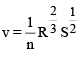Stickler's formula or Chezy's formula :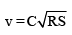Regime channels: Silting = Scouring.

Kutter's formula: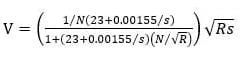Kennedy's theory:
RG Kennedy investigated canals systems for twenty years and come up with a Kennedy’s silt theory. The theory says that, the silt carried by flowing water in a channel is kept in suspension by the eddy current rising to the surface.
The vertical component of the eddy current tries to move sediment up whereas sediment weight tries to bring it down. Therefore, if adequate velocity available to create eddies so as to keep the sediment just in suspension silting will be prevented.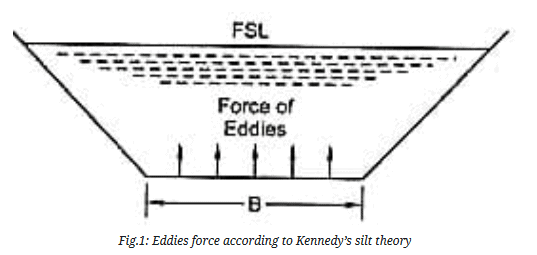Critical velocity based on Kennedy’s Silt Theory:
Critical velocity is the mean velocity which will just make the channel free from silting and scouring. The velocity is based on the depth of the water in the channel. The general form of critical velocity is as follow: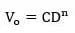Where,
V0 = Critical velocity
D = full supply depth
C & n: Constants which found to be 0.546 and 0.64, respectively.

Lacey’s theory:
Lacey stated that a channel may not be in regime condition even if it is flowing with non-scouring and non-silting velocity. Therefore, he distinguished three regime conditions as follows : (i) true regime (ii) initial regime (iii) final regime.
True regime:
A channel is said to be in regime condition if it is transporting water and sediment in equilibrium such that there is neither silting nor scouring of the channel.  But according to Lacey, the channel should satisfy the following conditions to be in regime condition.
-Canal discharge should be constant.
-The channel should flow through incoherent alluvium soil, which can be scoured as easily as it can be deposited and this sediment should be of the same grade as is transported.
-Silt charge, which is the minimum transported load should be constant.

If the above conditions are satisfied, then the channel is said to be in true regime condition. But this is not possible in actual practice.

Initial regime:
When only the bed slope of a channel varies and its c/s or wetted perimeter remains unaffected even then the channel can exhibit no silting, no scouring properties is called initial regime.

Final regime:
If there is no resistance from the sides and all the variables such as perimeter, depth, slope etc. are equally free to vary and finally get adjusted according to discharge and silt grade then the channel is said to have achieve permanent stability called final regime.

Lacey's theory:
According to lacey’s, the design procedure to build canal is as follows :
-Canal discharge (Q) and mean particle size (dm) should be known.
-From the mean size or diameter of the particle (dm), silt factor is first calculated using the below expression :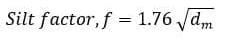Using discharge and silt factor, velocity (V) can be calculated by the expression as follows: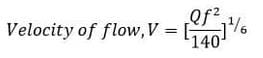After attaining the velocity of canal flow, find the area of the canal by dividing discharge with velocity. Also, find the mean hydraulic depth (R) of the canal and wetted perimeter (P) of the canal.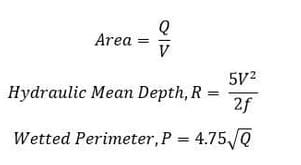Assume the bed slope (S) value or find by substituting the values of silt factor and canal discharge in the following formula :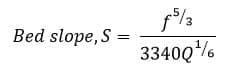Drawbacks of Lacey’s Silt Theory:
-Lacey did not explain the properties that govern the alluvial channel.
-In general, flow is different at bed and sides of the channel which requires two different  silt factors but Lacey derived only one silt factor.
-The semi-elliptical shape proposed by Lacey as the ideal shape of the channel is not convincing.
-Lacey did not consider the silt concentration in his equations.
-Attrition of silt particles is ignored by Lacey.
-Lacey did not give proper definitions for the silt grade and silt charge.

The document Distribution System - Notes | Study Civil Engineering SSC JE (Technical) - Civil Engineering (CE) is a part of the Civil Engineering (CE) Course Civil Engineering SSC JE (Technical).
All you need of Civil Engineering (CE) at this link: Civil Engineering (CE)

## Civil Engineering SSC JE (Technical)

2 videos|122 docs|50 tests
 Use Code STAYHOME200 and get INR 200 additional OFF

## Civil Engineering SSC JE (Technical)

2 videos|122 docs|50 tests

Track your progress, build streaks, highlight & save important lessons and more!

,

,

,

,

,

,

,

,

,

,

,

,

,

,

,

,

,

,

,

,

,

;